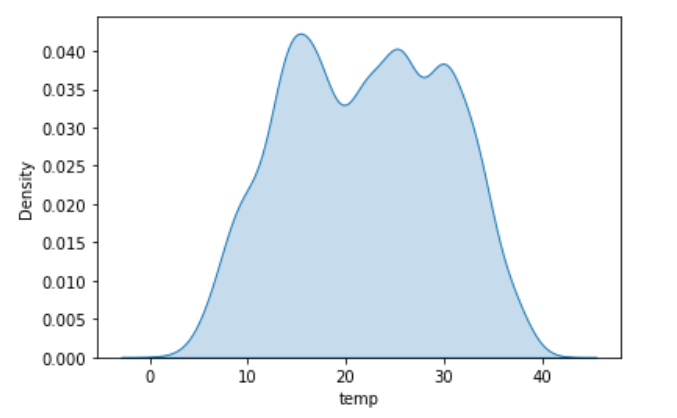# How to Make a Ridge Plot in Python

If you are like me, you have never heard of a ridge plot. However, I find that it’s one of the more interesting plots used to describe numerical data. It allows you display the distribution of data across a dimension. In other words, you will be able to see the distribution of temperatures across a month or the sales distribution across departments.

Let’s dive into the actual code and create a Ridge plot. First, we start with a distribution plot. A KDE plot, histogram or any other distribution plot are good examples to use If we evaluate temperatures in our bike dataset, we can the see distribution of temperatures across the entire year.

``````# distribution of temperatures across the year.
import pandas as pd
import seaborn as sns
sns.kdeplot(df['temp'])``````## Lets Prepare the Data for a Ridge

``````#isolate the year and create a month name
df_11 = df[df['year']==2011]
df_11['month'] = df_11['datetime'].dt.strftime('%B')
#lets map the mean for each month to the original data to get a nice color hue
df_11['mean_month'] = df_11['month'].map(df_11.groupby(['month'])['temp'].mean())``````

## Let’s Create the Visuals For the Ridge Plot

``````# Lets chose a color palette and save it as a variable.
pal = sns.color_palette(palette='coolwarm', n_colors=12)

# We use the hue to get the colors of by month we need to use the mean_month value that is going to be used to color each distribution
g = sns.FacetGrid(df_11, row='month', hue='mean_month', aspect=15, height=0.4, palette=pal)

# then we add the densities kdeplots for each month
g.map(sns.kdeplot, 'temp',
fill=True, alpha=1, linewidth=1.5)

# This adds the outline with black color which essentially the same graph just empty
g.map(sns.kdeplot, 'temp',
color="k", lw=2)

#This will add a line across each graph
g.map(plt.axhline, y=0,
lw=2, clip_on=False)

# Cycle through  axes add the month
in each matplotlib.Axes
for i, ax in enumerate(g.axes.flat):
ax.text(-15, 0.02, month_dict[i+1],
fontweight='bold', fontsize=15)

# This will allow you to create the overlap look

# eventually we remove axes titles, yticks and spines
g.set_titles("")
g.set(yticks=[])
g.despine(bottom=True, left=True)
g.set_xlabels("")
g.set_ylabels("")
plt.setp(ax.get_xticklabels(), fontsize=15, fontweight='bold')
plt.xlabel('Temperature in degree Celsius', fontweight='bold', fontsize=10)
g.fig.suptitle('Daily Average Temperature by Month',
fontsize=20,
fontweight=20)``````

Let’s look at some other examples of a ridge plot looking a video games sales. In this example, we will use a distplot from the Seaborn library instead of a KDEplot.

• in
• |
• December 10, 2022#### Gaelim Holland

Subscribe
Notify of